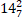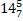# Quiz Discussion

In an exam, the average marks obtained by Jhon in English, Math, Hindi and Drawing were 50. His average mark in Maths, Science, Social Studies and Craft were 70. If the average mark in all seven subjects is 58, his score in Maths was -

Course Name: Quantitative Aptitude

• 1] 50
• 2] 52
• 3] 60
• 4] 74
##### Solution
No Solution Present Yet

#### Top 5 Similar Quiz - Based On AI&ML

Quiz Recommendation System API Link - https://fresherbell-quiz-api.herokuapp.com/fresherbell_quiz_api

# Quiz
1
Discuss

A man started his journey from Lucknow to Kolkata, which is 200 km, at the speed of 40 kmph then he went to Banglore which is 300 km, at the speed of 20 kmph. Further he went to Ahmedabad which is 500 km, at the speed of 10 kmph. The average speed of the man is :

• 1]kmph

• 2]Kmph

• 3]

15.6 kmph

• 4]

16.1 kmph

##### Solution
2
Discuss

The average of 20 numbers is zero. Of them, at the most, how many may be greater than zero ?

• 1]

0

• 2]

-1

• 3]

1

• 4]

None Of These

##### Solution
3
Discuss

The average weight of a class of 24 students is 35 kg. If the weight of the teacher be included, the average rises by 400 g. The weight of the teacher is -

• 1] 45 kg
• 2] 50 kg
• 3] 53 kg
• 4] 55 kg
##### Solution
4
Discuss

The average marks of four subjects is 120. If 33 was misread as 13 during the calculation, what will be the correct average?

• 1] 122
• 2] 120
• 3] 125
• 4] 121
##### Solution
5
Discuss

The arithmetic mean of the scores of a group of students in a test was 52. The brightest 20% of them secured a mean score of 80 and the dullest 25% a mean score of 31. The mean score of remaining 55% is-

• 1] 45
• 2] 50
• 3] 51.4 approx
• 4] 54.6 approx
##### Solution
6
Discuss

10 years ago, the average age of a family of 4 members was 24 years. Two children having been born (with age difference of 2 years), the present average age of the family is again 24 years. Then the present age of the youngest child is:

• 1]

1 years

• 2]

2 years

• 3]

3 years

• 4]

4 years

##### Solution
7
Discuss

The average of 6 observations is 45.5. If one new observation is added to the previous observations, then the new average becomes 47. The new observation is :

• 1] 58
• 2] 56
• 3] 50
• 4] 46
##### Solution
8
Discuss

If the average of 5 consecutive integers is x then, find the average of next to next 5 consecutive integers.

• 1] x + 5
• 2] x - 5
• 3] x + 10
• 4] x + 25
##### Solution
9
Discuss

In a primary school the average weight of male students is 65.9 kg and the average weight of female students is 57 kg. If the average weight of all the students ( both male and female ) is 60.3 kg and the number of male students in the school is 66, what is the number of female students in the school?

• 1] 162
• 2] 168
• 3] 180
• 4] 112
##### Solution
10
Discuss

The average of a group of men is increased by 5 years when a person aged of 18 years is replaced by a new person of aged 38 years. How many men are there in the group?

• 1] 3
• 2] 4
• 3] 5
• 4] 6
• 5] 7
# Quiz